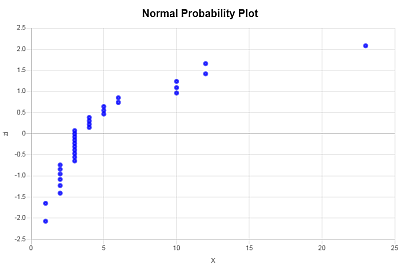# Normal Probability Plot Maker

Instructions: Use this Normal Probability Plot maker by entering the sample data below and this statistics calculator will provide step-by-step calculation of the required elements to construct the required probability plot.Type the sample (comma or space separated)Name of the variable (Optional)

## More About the Normal Probability Plot

A normal probability plot is a plot that is typically used to assess the normality of the distribution to which the passed sample data belongs to.

There are different types of normality plots (P-P, Q-Q and other varieties), but they all operate based on the same idea. The theoretical quantiles of a standard normal distribution are graphed against the observed quantiles.

Therefore, if the sample data comes from a normality distributed population , then the normal probability plot should look like a 45 o line, with random variations about it. If that is not the case, and the pattern of the normal probability plot departs significantly/systematically from the normal probability plot, then one should suspect that the distribution is not normal.

### How do you compute a normal probability plot?

There are several concrete steps you need to take, in a specific order to construct a normal probability plot

1. In this concrete case, the data are ordered in ascending order, and we call such data as $$X_1, X_2, ...., X_i , ...., X_n$$.
2. For each $$X_i$$ in this sequence of ordered data, we compute the theoretical frequencies $$f_i$$, which are approximated using the following formula:
3. $f_i = \frac{i - 0.375}{n + 0.25}$ (where $$i$$ corresponds to the position in the ordered dataset)
4. We then we also compute $$z_i$$, is corresponding associated z-score as
5. $z_i = \Phi^{-1}(f_i)$
6. Then, the normal probability plot is obtained by plotting the ordered X-values (your sample data) on the horizontal axis, and the corresponding $$z_i$$ values on your vertical axis.

### Normal probability plot Excel

You can plot a normal probability graph in Excel, but it takes some time. Yo

### Calculators for the normal distribution and others

Other chart makers you can use are our normal distribution grapher , scatter plot maker or our Pareto chart maker .

### Example: Calculation of a normal probability plot

Question: You are provided with the following sample data: 2, 3, 4, 3, 3, 2, 3, 4, 5, 3, 2, 3, 1, 2, 3, 4, 5, 6, 3, 2, 4, 5, 6 10 10 10 12 12 1 2 3 3 and 23. Construct a normal probability plot.

Solution:

We need to construct a normal probability plot. These are the sample data that have been provided:

 Observation: $$X$$ 1 2 2 3 3 4 4 3 5 3 6 2 7 3 8 4 9 5 10 3 11 2 12 3 13 1 14 2 15 3 16 4 17 5 18 6 19 3 20 2 21 4 22 5 23 6 24 10 25 10 26 10 27 12 28 12 29 1 30 2 31 3 32 3 33 23

The theoretical frequencies $$f_i$$ need to be computed as well as the associated z-scores $$z_i$$, for $$i = 1, 2, ..., 33$$:

Observe that the theoretical frequencies $$f_i$$ are approximated using the following formula:

$f_i = \frac{i - 0.375}{n + 0.25}$

where $$i$$ corresponds to the position in the ordered dataset, and $$z_i$$ is corresponding associated z-score. This iscomputed as

$z_i = \Phi^{-1}(f_i)$

The following table is obtained

 Position (i) X (Asc. Order) fi zi 1 1 0.0188 -2.079 2 1 0.0489 -1.656 3 2 0.0789 -1.412 4 2 0.109 -1.232 5 2 0.1391 -1.084 6 2 0.1692 -0.957 7 2 0.1992 -0.844 8 2 0.2293 -0.741 9 3 0.2594 -0.645 10 3 0.2895 -0.555 11 3 0.3195 -0.469 12 3 0.3496 -0.386 13 3 0.3797 -0.306 14 3 0.4098 -0.228 15 3 0.4398 -0.151 16 3 0.4699 -0.075 17 3 0.5 0 18 3 0.5301 0.075 19 4 0.5602 0.151 20 4 0.5902 0.228 21 4 0.6203 0.306 22 4 0.6504 0.386 23 5 0.6805 0.469 24 5 0.7105 0.555 25 5 0.7406 0.645 26 6 0.7707 0.741 27 6 0.8008 0.844 28 10 0.8308 0.957 29 10 0.8609 1.084 30 10 0.891 1.232 31 12 0.9211 1.412 32 12 0.9511 1.656 33 23 0.9812 2.079

The normal probability plot is obtained by plotting the X-values (your sample data) on the horizontal axis, and the corresponding $$z_i$$ values on your vertical axis. The following normality plot is obtained: